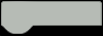# Project 5 Colorful RGB LEDIn this chapter let’s try this colorful RGB LED. It combines red, green and blue three colors to produce over 16 million hues of light. In this project, we are gonna make an RGB LED generate different colors randomly.Please figure out whether your RGB LED is common cathode or common anode before connecting. If you don’t know how to determine, jump to hardware review part to learn. Pay attention to the pin order.Input the example code 5-1. We need to create a new block. Click “Add an Input” to name the function.Drag out the “red” to use it as a variable. It is a local variable and can be called only in this function.Example Program 5-1:Upload the codes into Arduino Board, then we can see that the LED changes constantly in random color.Code Learning

CODE
``````1.// function declaration
2.void colorRGB(float red, float green, float blue);
3.
4.
5.// main program starts
6.void setup() {
7.     dfrobotRandomSeed();
8.}
9.void loop() {
10.    colorRGB((random(0, 255+1)), (random(0, 255+1)), (random(0, 255+1)));
11.    delay(1000);
12.}
13.
14.
15.// define function
16.void colorRGB(float red, float green, float blue) {
17.    analogWrite(9, (constrain(red, 0, 255)));
18.    analogWrite(10, (constrain(green, 0, 255)));
19.    analogWrite(11, (constrain(blue, 0, 255)));
20.}  ``````

The RGB LED is a combination of 3 LEDs in just one package, so we need 3 PWM pins to control the RGB LED.

The most important part, the main function, has a function colorRGB() with three parameters to be input. They are used to write value into red, green and blue. When we want to use a color in the program, we can assign a value to them directly.

There are two new functions constrain() and random(). Try looking them up with the websites we mentioned in the last chapter.There are three parameters in the constrain(): x, a and b. x is the number to constrain; a is the lower end of the range; b is the upper end of the range.

Returns

x: if x is between a and b.

a: if x is less than a.

b: if x is greater than b.

In the program, the red, green and blue are constrain within 0~255 (the range of PWM).

￭ dfrobotRandomSeed();

The function dfrobotRandomSeed() is used to set random seed, a random number generator. Read the uncertain noise in air via the analog pin (A6, A7), use it as random seed and then we can get an ideal random number.

￭ random (min,max)

random() is used to generate a random, min is the lower bound of the random value, and max is the upper bound. To ensure the number “255” can be picked in Mind+, the maximum 255 in the auto-generated code is written as “255+1”.RGB LED

RGB LEDs have four pins—one for each LED and another for the common anode or cathode. The RGB LED we use here is common cathode. In a common cathode RGB LED, all three LEDs share a negative connection(cathode), while in a common anode RGB LED, the three LEDs share a positive connection(anode). The figure below illustrates how three LEDs change into an RGB LED.How to use an RGB LED? How to create different colors?

An RGB LED is actually three LEDs, red, green and blue inside one package. By configuring the intensity of each LED via the PWM port on Arduino(analogWrite()), you can produce any colors you want.We can configure 256*256*256(1677216) kinds of colors by assigning different PWM to these three LEDs.

Distinguish between RGB LED common anode and common cathode

What’s the difference between common anode and common cathode? From the figure below, we could see that they have similar appearance.The best way to distinguish them is by using a multimeter. Put your multimeter in continuity mode.

￭ If the LED lights up with the red multimeter tip on the longest lead and the black on one of the other leads--you have a common anode RGB LED.

￭ If the LED lights up with the black multimeter tip on the longest lead and the red on one of the other leads--you have a common cathode RGB LED.1.Input the codes of this project into Mind+ Manually, compile and debug.

2.Based on the colorful RGB LED, change the codes to make a Rainbow RGB light. Use the function colorRGB() and adjust the value of red, blue and green in 0-255 to create the color you want.

3.Combine the breathing LED and Rainbow light together to display a smooth rainbow lighting effect.